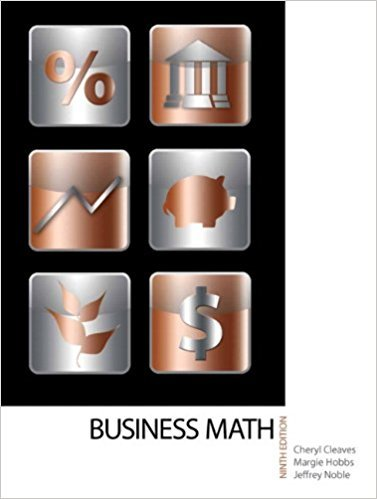×
×

# Solutions for Chapter 13-1: COMPOUND INTEREST AND FUTURE VALUE## Full solutions for Business Math, | 9th Edition

ISBN: 9780135108178Solutions for Chapter 13-1: COMPOUND INTEREST AND FUTURE VALUE

Solutions for Chapter 13-1
4 5 0 246 Reviews
23
3
##### ISBN: 9780135108178

Chapter 13-1: COMPOUND INTEREST AND FUTURE VALUE includes 24 full step-by-step solutions. This expansive textbook survival guide covers the following chapters and their solutions. Since 24 problems in chapter 13-1: COMPOUND INTEREST AND FUTURE VALUE have been answered, more than 17422 students have viewed full step-by-step solutions from this chapter. This textbook survival guide was created for the textbook: Business Math, , edition: 9. Business Math, was written by and is associated to the ISBN: 9780135108178.

Key Math Terms and definitions covered in this textbook
• Big formula for n by n determinants.

Det(A) is a sum of n! terms. For each term: Multiply one entry from each row and column of A: rows in order 1, ... , nand column order given by a permutation P. Each of the n! P 's has a + or - sign.

• Echelon matrix U.

The first nonzero entry (the pivot) in each row comes in a later column than the pivot in the previous row. All zero rows come last.

• Ellipse (or ellipsoid) x T Ax = 1.

A must be positive definite; the axes of the ellipse are eigenvectors of A, with lengths 1/.JI. (For IIx II = 1 the vectors y = Ax lie on the ellipse IIA-1 yll2 = Y T(AAT)-1 Y = 1 displayed by eigshow; axis lengths ad

• Fourier matrix F.

Entries Fjk = e21Cijk/n give orthogonal columns FT F = nI. Then y = Fe is the (inverse) Discrete Fourier Transform Y j = L cke21Cijk/n.

• Hessenberg matrix H.

Triangular matrix with one extra nonzero adjacent diagonal.

• Independent vectors VI, .. " vk.

No combination cl VI + ... + qVk = zero vector unless all ci = O. If the v's are the columns of A, the only solution to Ax = 0 is x = o.

• Left nullspace N (AT).

Nullspace of AT = "left nullspace" of A because y T A = OT.

• Linearly dependent VI, ... , Vn.

A combination other than all Ci = 0 gives L Ci Vi = O.

• Markov matrix M.

All mij > 0 and each column sum is 1. Largest eigenvalue A = 1. If mij > 0, the columns of Mk approach the steady state eigenvector M s = s > O.

• Multiplicities AM and G M.

The algebraic multiplicity A M of A is the number of times A appears as a root of det(A - AI) = O. The geometric multiplicity GM is the number of independent eigenvectors for A (= dimension of the eigenspace).

• Normal matrix.

If N NT = NT N, then N has orthonormal (complex) eigenvectors.

• Orthogonal subspaces.

Every v in V is orthogonal to every w in W.

• Pivot.

The diagonal entry (first nonzero) at the time when a row is used in elimination.

• Rank r (A)

= number of pivots = dimension of column space = dimension of row space.

• Similar matrices A and B.

Every B = M-I AM has the same eigenvalues as A.

• Simplex method for linear programming.

The minimum cost vector x * is found by moving from comer to lower cost comer along the edges of the feasible set (where the constraints Ax = b and x > 0 are satisfied). Minimum cost at a comer!

• Spectral Theorem A = QAQT.

Real symmetric A has real A'S and orthonormal q's.

• Toeplitz matrix.

Constant down each diagonal = time-invariant (shift-invariant) filter.

• Trace of A

= sum of diagonal entries = sum of eigenvalues of A. Tr AB = Tr BA.

• Tridiagonal matrix T: tij = 0 if Ii - j I > 1.

T- 1 has rank 1 above and below diagonal.

×